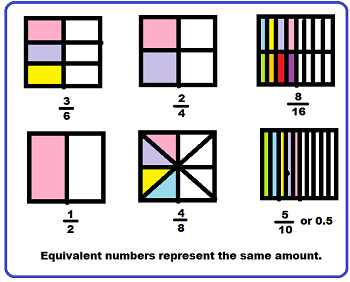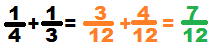# What Does Equivalent Mean in Math?

An error occurred trying to load this video.

Try refreshing the page, or contact customer support.

Coming up next: 1st Grade Math Vocabulary: Lesson for Kids

### You're on a roll. Keep up the good work!

Replay
Your next lesson will play in 10 seconds
• 0:03 Equal Pieces of Cake
• 0:46 Equivalent Numbers
• 2:29 Converting Decimals…
• 3:33 Lesson Summary
Save Save

Want to watch this again later?

Timeline
Autoplay
Autoplay
Speed Speed

#### Recommended Lessons and Courses for You

Lesson Transcript
Instructor
Bethany Calderwood

Bethany has taught special education in grades PK-5 and has a master's degree in special education.

Expert Contributor
Laura Pennington

Laura received her Master's degree in Pure Mathematics from Michigan State University. She has 15 years of experience teaching collegiate mathematics at various institutions.

Equivalent numbers are used to make different math problems easier to complete. In this lesson, you'll learn the meaning of equivalent numbers, as well as how to find some of your own.

## Equal Pieces of Cake

Mom made mini cakes for dessert. She gave Chris one-half of a cake, Leila three-sixths of a cake, and Jim four-eighths of a cake. Chris said it wasn't fair because Jim got four pieces of cake, while he only got one. Leila said it wasn't fair because Chris' half of the cake was bigger than her three pieces. Jim said he thought all their portions were the same. Who was right?Look at this picture. One half, three-sixths, and four-eighths are all fractions that show the same amount of cake! The picture shows that two-fourths, eight-sixteenths, and five-tenths are also equal to the same amount of cake. This is because all of the portions are equivalent fractions.

## Equivalent Numbers

Equivalent means equal in value, function, or meaning. In math, equivalent numbers are numbers that are written differently but represent the same amount. In the picture of the boxes, the same amount of each box is colored in, but because each box is divided into a different number of sections, the number is written differently.

### Equivalent Fractions

Equivalent fractions can be found by multiplying both the numerator (top number) and denominator (bottom number) by the same number. They can also be found by reducing a fraction to its simplest terms.

In order to add or subtract fractions, they need to have like denominators. That means the fractions must have the same number on the bottom of each fraction. To add or subtract fractions, it's often necessary to use equivalent fractions. Look at this problem.As they stand now, the fractions 1/4 and 1/3 cannot be added, but as equivalent fractions of 3/12 and 4/12, they can be added.

### Equivalent Decimals

When you're working with decimals, sometimes it is helpful to add a zero as a placeholder. This is okay if the zero is placed to the right of the decimal point after the last number. A decimal and a zero can also be added after a whole number, which is often done to facilitate long division and multiplication. Here are a few examples of equivalent decimals, or decimals that have the same value:

• 3.4 = 3.40
• 5 = 5.0
• 2.62 = 2.620

These are not equivalent because adding a zero changes the value of the number:

• 3.4 and 3.04
• 9.4 and 90.4
• 4.55 and 4.505

To unlock this lesson you must be a Study.com Member.

## Equivalent Numbers Bingo Game

### Reminder:

• Equivalent numbers are numbers that have the same value.
• Each type of number, such as fractions, decimals, or square roots, can be equivalent to other numbers of their types, or to numbers of different types, as long as they have the same value.

### Materials Needed:

• Bingo cards with various numbers on them in a rectangular pattern
• Bingo chips
• A bucket or container of numbers on pieces of paper, such that the numbers on the bingo cards are equivalent to these numbers.

### Directions and Rules:

1. Hand out bingo cards and bingo chips to each player. This can be played alone or with a group. However many bingo cards you have is the number of people that can play.
2. Pull a number out of the number bucket.
3. Each player places a bingo chip on any number on their card that is equivalent to the number pulled from the bucket.
4. This continues until someone gets a row, column, or diagonal of chips on their card. At this point, the person with a full row, column, or diagonal says "equivalent numbers bingo".
5. Whoever gets a correct equivalent numbers bingo first wins the game.

### Sample Round of One Number Being Pulled From the Number Bucket

Gary and Fiona are playing equivalent numbers bingo. The first number pulled from the bucket is 0.2. Fiona sees that she has the number √(0.04) and the number 2/10 on her card. First, she looks at √(0.04). She knows that 22 = 4, so she sees a possible connection between 0.2 and √(0.04), and decides to calculate √(0.04).

• √(0.04) = 0.2

Sure enough, she finds that √(0.04) = 0.2, so she knows that these two numbers are equivalent, and she places a chip over √(0.04). She then considers the number 0.2 as a fraction. Since the 2 is in the tenths place, the number is read as two-tenths, so she knows that the fraction, 2/10, is equivalent to 0.2, so she places a chip over that number as well.

Gary is looking at his card, and doesn't see anything that looks equivalent to 0.2, but he knows that if he performs the division indicated by the fractions on his card, then he could find that they actually do have the same value as 0.2. There are two fractions on his card; 3/8 and 1/5, so he performs the division for each of these.

• 3 ÷ 8 = 0.375
• 1 ÷ 5 = 0.2

He sees that 3/8 has a decimal value of 0.375, so this is not equivalent to 0.2. However, he also sees that 1/5 has a decimal value of 0.2, so this is equivalent to 0.2, and he places a chip over 1/5. After this, the next number is pulled from the bucket, and they play until one of them gets an equivalence number bingo.

### Register to view this lesson

Are you a student or a teacher?

#### See for yourself why 30 million people use Study.com

##### Become a Study.com member and start learning now.
Back
What teachers are saying about Study.com

### Earning College Credit

Did you know… We have over 200 college courses that prepare you to earn credit by exam that is accepted by over 1,500 colleges and universities. You can test out of the first two years of college and save thousands off your degree. Anyone can earn credit-by-exam regardless of age or education level.